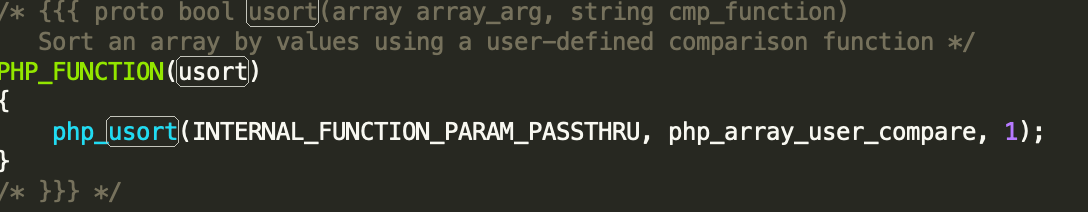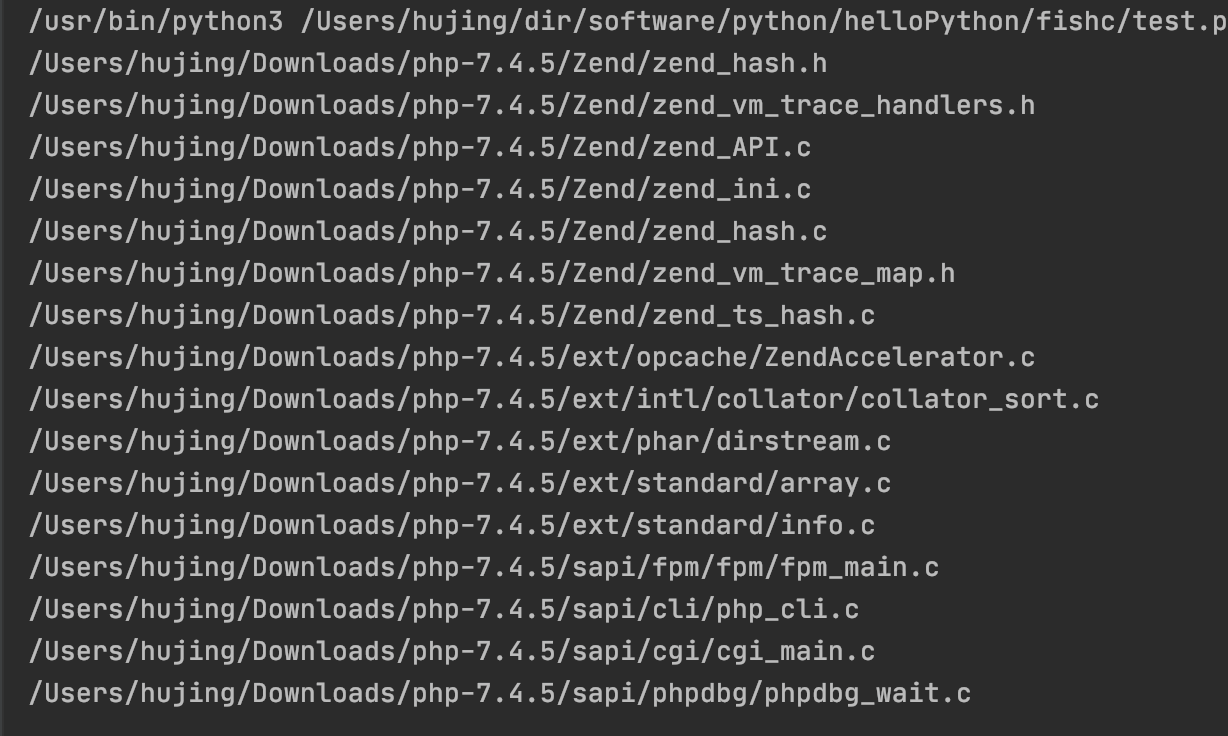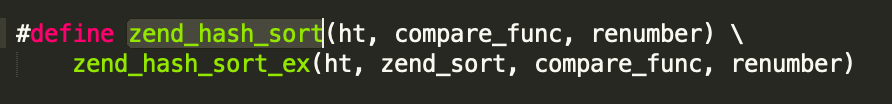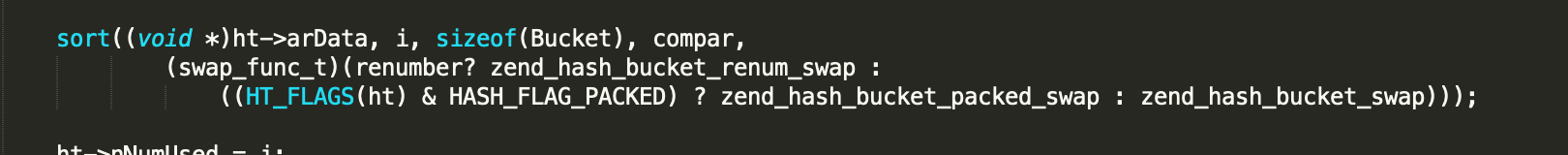# 引出

usort($arr, function ($a, $b){ // 这里添加了 order 字段, 默认为0, 将order大的提到前边 return$b['order'] - $a['order']; }); 但是, 今天我大哥突然告诉我, php的usort是不稳定的, 也就是在两个元素相等的情况下, 不能够保证两个元素的位置不变. 在我想到的排序算法中: 选择, 冒泡, 插入, 快排, 希尔, 堆排, 计数, 归并, 其中可以稳定排序的算法有: 冒泡, 插入, 归并. 而这几个算法, 时间复杂度较小的是: 快排, 归并, 堆排. 时间复杂度是O(log n). 如果要选择一款既能够保证稳定性, 时间复杂度又小的算法, 二者取交集也得选择 归并 吧. 但是, 毕竟我不是PHP作者, 咱也不知道人家到底用的是什么, 于是乎, 我决定实验一下, 下面这段代码产生了: // 生成一个随机数组$arr = [];
for ($j = 0;$j < 100; $j++){$arr[] = [
'id' => $j, 'order' => random_int(0, 3), ]; } // 按照order进行排序 usort($arr, function ($a,$b){
return $b['order'] -$a['order'];
});
// 验证是否稳定
$lastValue = null; foreach ($arr as $value){ if(empty($lastValue)){
$lastValue =$value;
continue;
}
// 若当前元素与上一个元素order相同, 判断
if($value['order'] ==$lastValue['order']){
// 当前不稳定了, 把数组打印出来
if($value['id'] <$lastValue['id']){
echo '不稳定', PHP_EOL;
exit;
}
}
$lastValue =$value;
}

# 分析static void php_usort(INTERNAL_FUNCTION_PARAMETERS, compare_func_t compare_func, zend_bool renumber) /* {{{ */
{
zval *array;
zend_array *arr;
zend_bool retval;
PHP_ARRAY_CMP_FUNC_VARS;

PHP_ARRAY_CMP_FUNC_BACKUP();

// 这个 zend 打头的函数一看就是虚拟机相关的, 不管了
ZEND_PARSE_PARAMETERS_START(2, 2)
Z_PARAM_ARRAY_EX2(array, 0, 1, 0)
Z_PARAM_FUNC(BG(user_compare_fci), BG(user_compare_fci_cache))
ZEND_PARSE_PARAMETERS_END_EX( PHP_ARRAY_CMP_FUNC_RESTORE(); return );

arr = Z_ARR_P(array);
// 一看就是对数组进行判空, 跳过
if (zend_hash_num_elements(arr) == 0)  {
PHP_ARRAY_CMP_FUNC_RESTORE();
RETURN_TRUE;
}

/* Copy array, so the in-place modifications will not be visible to the callback function */
arr = zend_array_dup(arr);

// 真正进行排序的方法
retval = zend_hash_sort(arr, compare_func, renumber) != FAILURE;

// 一些善后操作
zval_ptr_dtor(array);
ZVAL_ARR(array, arr);

PHP_ARRAY_CMP_FUNC_RESTORE();
RETURN_BOOL(retval);
}1. 若数组长度小于等于16, 使用 插入排序
2. 若数据长度大于16, 使用 快速排序 (快速排序对元素个数1024前后做了不同的处理, 应该是优化)

# 总结

If you want to keep the order when two members compare as equal, use this.
<?php

function stable_uasort(&$array,$cmp_function) {
if(count($array) < 2) { return; }$halfway = count($array) / 2;$array1 = array_slice($array, 0,$halfway, TRUE);
$array2 = array_slice($array, $halfway, NULL, TRUE); stable_uasort($array1, $cmp_function); stable_uasort($array2, $cmp_function); if(call_user_func($cmp_function, end($array1), reset($array2)) < 1) {
$array =$array1 + $array2; return; }$array = array();
reset($array1); reset($array2);
while(current($array1) && current($array2)) {
if(call_user_func($cmp_function, current($array1), current($array2)) < 1) {$array[key($array1)] = current($array1);
next($array1); } else {$array[key($array2)] = current($array2);
next($array2); } } while(current($array1)) {
$array[key($array1)] = current($array1); next($array1);
}
while(current($array2)) {$array[key($array2)] = current($array2);
next(\$array2);
}
return;
}0 评论

0